This content got low rating by people.

Tokens power day 10 10/09/2021 part 1

The purpose of this account will be to store Hives as well as tokens (tokens in power)

Objectif :

Alive + 2.509
total = 143.326
Objectif = 1 000
vote value = 0.079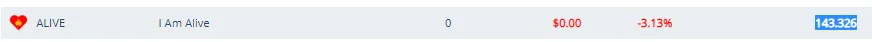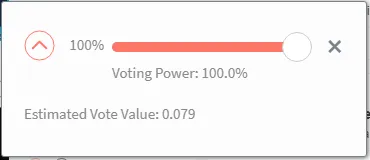Neoxag + 248.39484102
total = 463.35368777
Objectif = 1 000
vote value = 0.01066882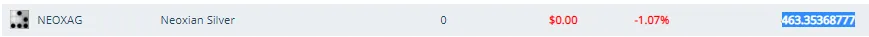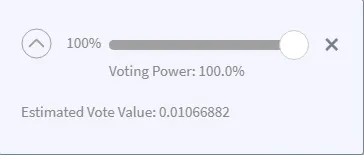Cine + 0.55760421
total = 2.81907591
Objectif = 1 000
vote value = 0.00136074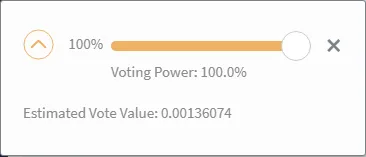BHT + 1.09329
total = 33.23082
Objectif = 1 000
vote value = 0.06178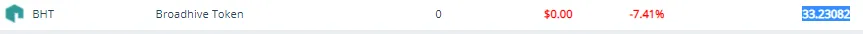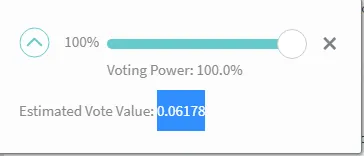Leo + 0.006
total = 1.319
Objectif = 1 000
vote value = NAN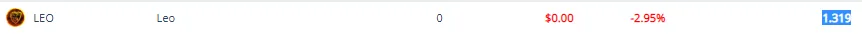build + 0.002
total = 0.176 ( 10.8293504 )
Objectif = 1 000
vote value = 0.00018785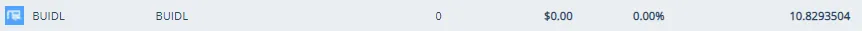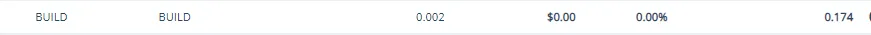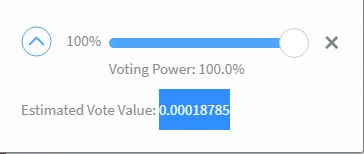Lern + 13.81596961
total = 128.3585088
Objectif = 1 000
vote value = 0.55979528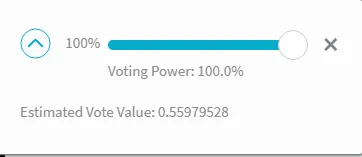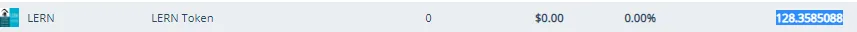Creative coin + 0.81276
total = 12.388
Objectif = 1 000
vote value = 0.00214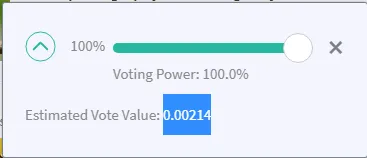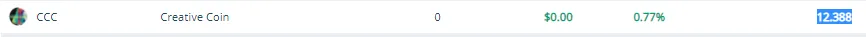0.01043622

Archon + 0.01043622
total = 0.04539584
Objectif = 1 00
vote value = ???????